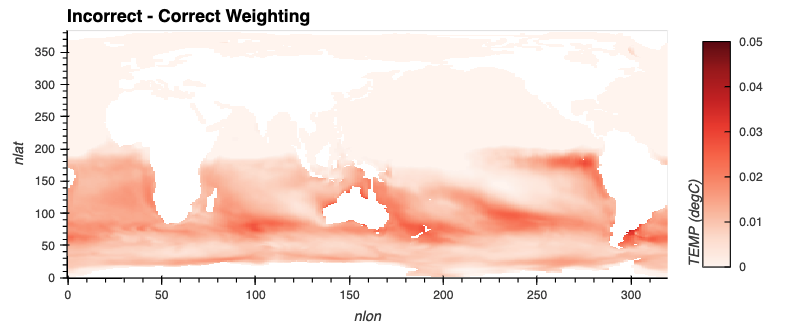# Correctly Calculating Annual Averages with Xarray#

A common component of people’s workflows is calculating annual averages, which helps reduce the frequency of datasets, making them easier to work with. Two of the data frequencies you may be looking to convert to annual include:

• Daily (365 days in each year)

• Monthly (12 months in a year)

## The Data#

When using the daily data, calculating the averages is relatively straightforward since we do not have to do any weighting, taking the length of each time into account. We know that each day is equal in length, and there are 365 days in each year.

This is not the case with monthly data. When converting monthly frequency into annual frequency, we need to take the length of each month into account since not each month is created equal. For example, February has 28 days whereas December has 31 - we need to make sure to get the weights right.

In this example, we will be using monthly data from the CESM2-Large Ensemble which is stored on AWS.

## The Problem#

Within Xarray, it can be tempting to use the resample or groupby functions to calculate your annual average, but you need to be careful here! By default these functions do not take the weight of the frequencies into account. We need to write a specialized workflow to account for this!

Here is a preview of how far off two different, seemingly similar methods of calculating annual averages with Xarray are!## The Solution#

Let’s dig into our solution - we will start by computing the yearly average from monthly data using resample, which is considered the “incorrect” method. Then, we will provide an example of calculating the proper weights, and applying these to our “correct” weighted average.

### Imports#

We use some typical libraries (Xarray, Numpy, and Dask), along with some visualization packages (hvPlot and holoviews).

import holoviews as hv
import hvplot
import hvplot.xarray
import numpy as np
import xarray as xr
from distributed import Client
from ncar_jobqueue import NCARCluster

hv.extension('bokeh')